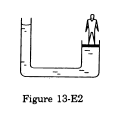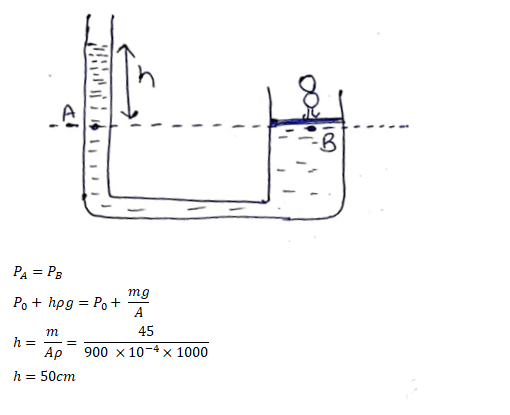# The area of cross-section of the wider tube shown in figure (13-E2)

Question:

The area of cross-section of the wider tube shown in figure (13-E2) is $900 \mathrm{~cm}^{2}$. If the boy standing on the piston weighs $45 \mathrm{~kg}$, find the difference in the levels of water in the two tubes.Solution: